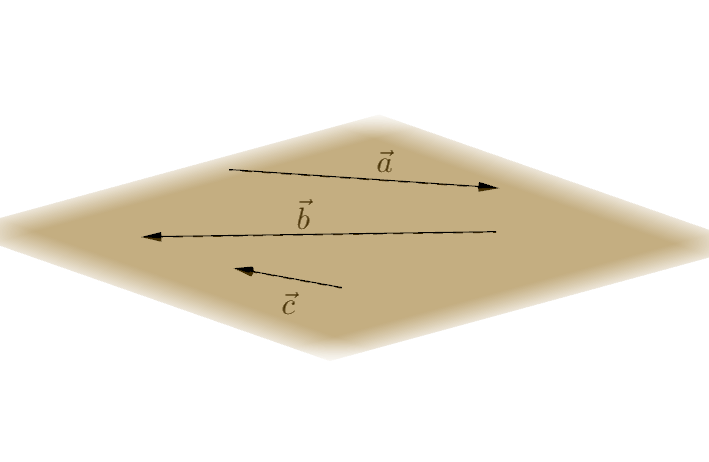Math Vector algebra Linear combination

# Linear combination

A linear combination of vectors is the addition of vectors, which are multiplied by a real number (scalar multiplication). This creates a new vector.

$\vec{v}=r_1\cdot\vec{a_1}+r_2\cdot\vec{a_2}+$ $...+r_n\cdot\vec{a_n}$

## Collinear vectors

Two vectors with parallel arrows are called collinear. One vector can then be represented as a linear combination of the other.$\vec{a}=r\cdot\vec{b}$ or $\vec{b}=r\cdot\vec{a}$

## Coplanar vectors

Vectors that can be depicted in the same plane are coplanar. In that case, each vector can be depicted as a linear combination of the other one.$\vec{a}=r\cdot\vec{b}+s\cdot\vec{c}$ or
$\vec{b}=r\cdot\vec{a}+s\cdot\vec{c}$ or
$\vec{c}=r\cdot\vec{a}+s\cdot\vec{b}$
!

### Remember

Collinear and coplanar vectors are also referred to as linearly dependent.